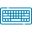# Power Series Calculator## Table of Content

Feedback

The power series calculator is designed to find the power series expansion by showing the functions as an infinite sum of functions.

## What is a Power Series?

In math analysis:

“The indication of infinite series for a given function is known as power series”.

## Power Series Formula:

Function to power series calculator finds the infinite series of forms and up to certain orders, it gives a plot of approximation of x by using the following formula:

$${\sum\limits_{n = 1}^\infty {{a_n}{x^n}} } = {{a_0} + {a_1}x }+{ {a_2}{x^2} + \ldots }+{ {a_n}{x^n} + \ldots }$$

A series containing the factor $$\left(x – x_{0} \right)$$ can also be considered for which the power series representation is given as follows:

$${\sum\limits_{n = 1}^\infty {{a_n}{{\left( {x – {x_0}} \right)}^n}} }= {{a_0} + {a_1}\left( {x – {x_0}} \right) }+{ {a_2}{\left( {x – {x_0}} \right)^2} +\ldots }+ {{a_n}{\left( {x – {x_0}} \right)^n} + \ldots ,}$$

Where:

• a is a coefficient of terms
• n is the maximum number of terms

## How To Find The Power Series?

It is the sum of terms of general form and In the power series the variable that is used is “x”. So, the converge or diverge value depends on the x value.

### Example:

Suppose the following power series term needs to evaluate by the radius of convergence.

$$\sum_{n=1}^\infty\frac{\left(x-9\right)^{n}}{n}$$

#### Solution:

Insert the ratio test in order to display the infinite series of functions.

$$C_{n}=\frac{\left(x-9\right)^{n}}{n} \hspace{0.25in} \text{Supposition}$$

The series will converge within a second if we take into service the power series solution calculator but here we find it manually.

$$L= \lim_{n \to \infty}\frac{\left(x-9\right)^{n}}{n}$$

$$L= \lim_{n \to \infty}[\frac{\left(x-9\right)^{n+1}}{n+1}* \frac{n}{\left(x-9\right)^n}]$$

$$L= \lim_{n \to \infty}[\frac{\left(x-9\right)^{∞+1}}{∞+1}* \frac{∞}{\left(x-9\right)^∞}]$$

$$L=\lim_{n \to \infty}[\frac{\left(x-9\right)^{1}}{1}* \frac{∞}{\left(x-9\right)}]$$

$$\left|x-9\right|$$

• Power series will converge for x - 9 < 1
• Power series will diverge for x - 9 > 1

For verification use the power series expansion calculator and get the answer within a fraction of a second.

## Working of Power Series Calculator:

The power series representation calculator with steps takes into account some values to show the power series expansion. Focus on these values.

Input:

• Put the value of the function
• Choose the type of variable
• Set the value of entering point
• Insert the value of the maximum order of the term
• Press on “Calculate”

Output:

Below are the results you will receive when using our power series finder.

• Power series expansion of a function
• Step-by-step calculations

## Ratio of Tests and Radius of Convergence:

The method to find out the various factors of power series is their ratios like;

• Convergence of infinite series
• Divergence of infinite series
• Intervals of convergence and divergence

### Ratio of Test Formula:

The power series calculator is used to investigate the ratio of the test. For a manual process the ratio formula is as follows:

$$L=\lim_{n \to \infty} \frac{a_{n+1}} {a_n}$$

“The distance that is drawn from the middle of the convergent series to the end”.

In the radius of convergence, the power series always converges at its center and finds a power series representation for the function calculator

## FAQs:

### Which Function Is Not a Power Function?

An exponential function is not a power function. It is a function in which the constant is raised to the power of the argument.

### Is The Power Series a Tylor Series?

In some smooth functions, every power series is a Tylor series. It is the series in which an infinite sum of terms is expressed as functions and is used to thepartial sum of approximate functions.

### Can a Power Series Be Multiplied?

Yes! As a polynomial multiplication, it also is multiplied.

## References:

From the source Wikipedia: Power series, Radius of convergence, Operations on power series, Analytic functions.### Sarah Taylor

I am a professional Chemist/Blogger & Content Writer. I love to research chemistry topics and help everyone learning Organic & Inorganic Chemistry and Biochemistry. I would do anything to spend vacations on a Hill Station.